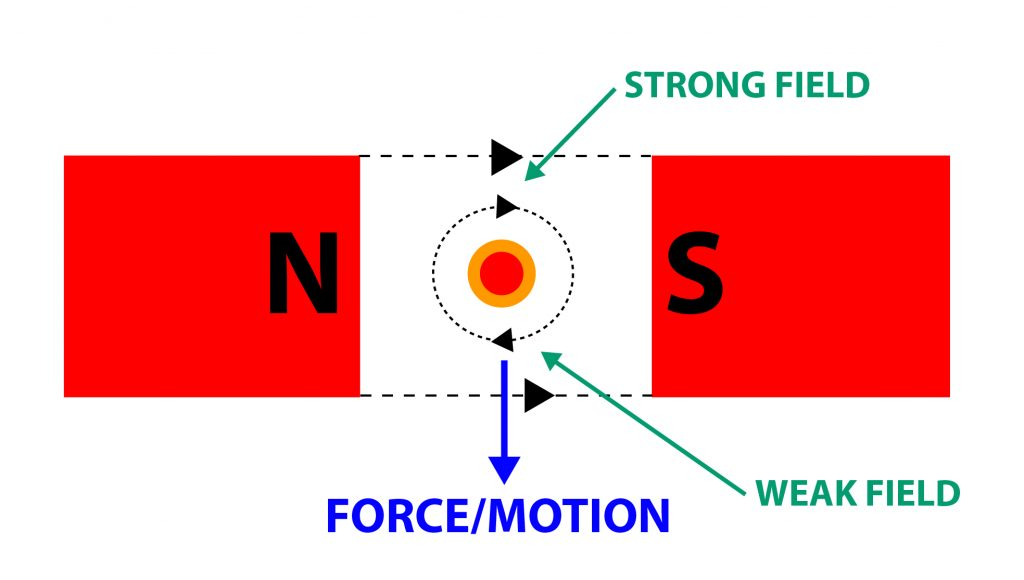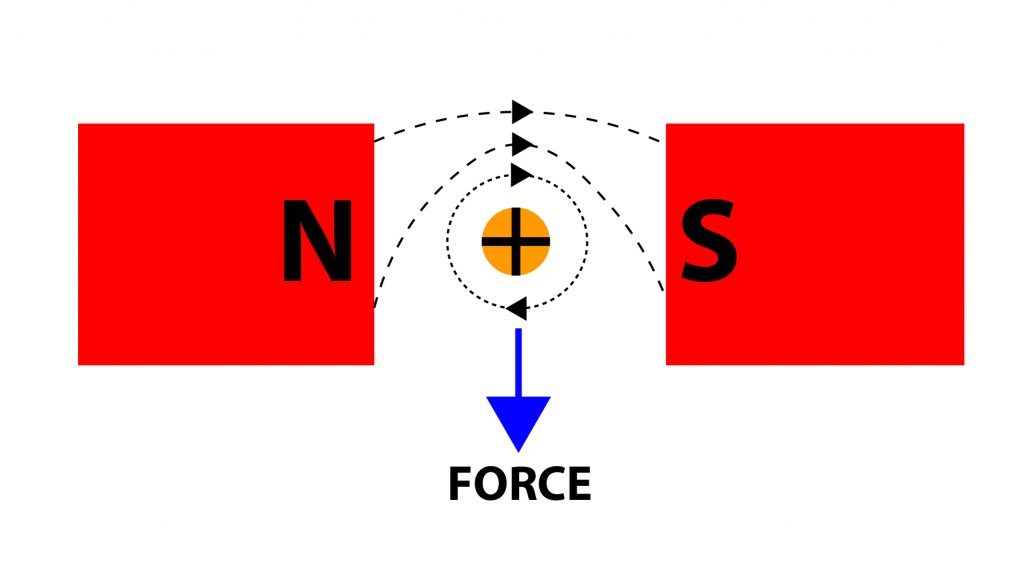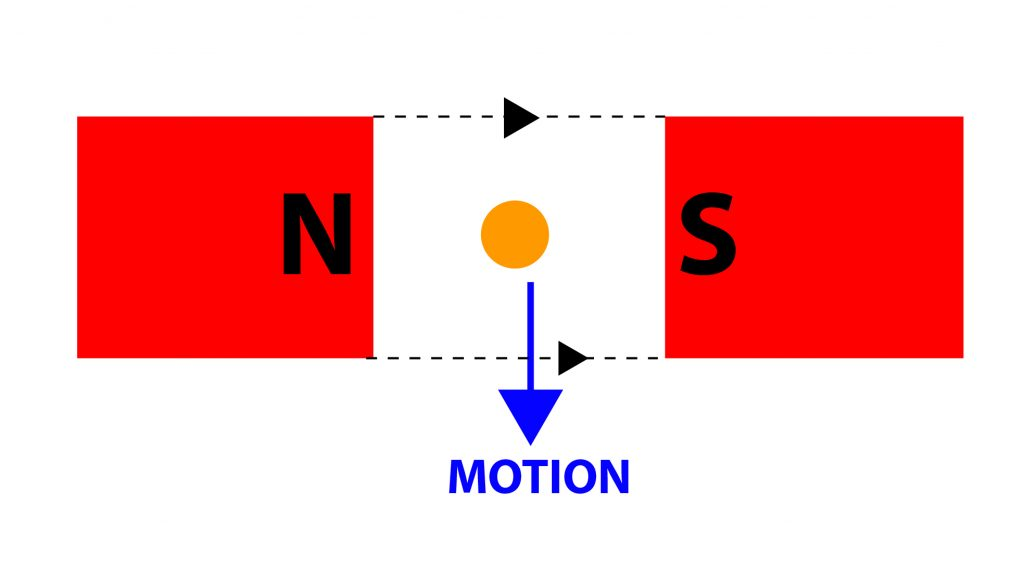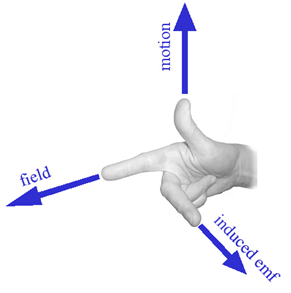Science

# The basic principles of electromagnetism

This article will provide a basic introduction to the principles of electromagnetism and electric motors.

As the name suggests, electromagnetism is a branch of physics that focuses on the interaction between electricity and magnetism. It plays a major role in most objects encountered in daily life. Electromagnetism is the interaction between conductors and fixed magnetic fields.

This video provides an excellent overview of magnetic fields, flux density and motor effect.

## What are the effects of magnetism?

All magnets have a north pole and a south pole. Like poles repel but opposite poles attract each other. Electrons in the atoms of magnets spin around the nucleus mostly in one direction, this is how the two poles are created. The magnetic force flows from the north pole to the south pole of the magnet. Connecting the north pole to the south pole of a different magnet (or vice versa) makes all the electrons spin in the same direction.

## What is the difference between magnetic flux and flux density?

Around a standard magnet there is a magnetic field which gives a flow of magnetic energy. This is known as magnetic flux (Φ) and is measured in Webers (Wb). Magnetic flux flows from the north pole of a magnet around to its south pole. The amount of magnetic flux flowing through a given area changes from one point to another around the magnet. Magnetic flux density is the amount of magnetic flux at a particular point, taken at a 90 degree angle to the magnetic flux’s direction. This measure is taken in Teslas (T)

## Motor principle and Fleming’s left-hand rule

Whenever a current carrying conductor lies in a magnetic field, there will be a force set up on that conductor.The direction of force on a current carrying conductor can be remembered by using Fleming’s left-hand rule.Fleming’s left-hand rule is the easiest way to remember the direction of motion in an electric motor. When current flows through a conducting wire and an external magnetic field is applied across that flow, the conducting wire experiences a force perpendicular (ie at a 90-degree angle) to both that field and the direction of the current flow. By using the three fingers of the left hand as in the diagram it is possible to see the three axes.

The thumb = the direction of force on the conductor.

The forefinger = the direction of the field flux.

The second finger = the direction of the current.

In practice, the main field will distort and the conductor will be pushed downwards.This is because:

•         Lines of magnetic flux that have the same direction push each other apart.
•         Lines of magnetic flux are in a permanent state of contraction.
•         Lines of magnetic flux do not cross.

Force is measured in Newtons (N). The magnitude of force depends on the strength of the main field, the strength of the field produced by the current carrying conductor and its active length.

The formula to remember for this is:

F = B x I x L

As an example, a conductor carrying a current of 2A and with an active length of 0.1m lies in a magnetic field with a flux density of 2.5T. The force on the conductor therefore is:

2.5 x 2 x 0.1 = 0.5N

What is Generator Principle?

Whenever a conductor moves through a magnetic field, there will be an induced EMF set up in that conductor.What is Lenz’s Law?

We can use Lenz’s Law to determine the direction of the current flow set up by the induced EMF. Lenz’s Law says that the induced EMF will set up an opposing force to the force creating it.

Eg:## Direction of motion and Fleming’s right-hand rule

The easiest way to remember the direction of motion in an electric generator is using Fleming’s right-hand rule. A different hand needs to be used for generators because of the differences between cause and effect in the two cases – with a motor the electric current and magnetic field exist (the causes) and lead to the force that creates the motion (the effect). In a generator the motion and magnetic field exist (causes) and lead to the creation of an electric current (the effect).

So in the right-hand rule:The thumb points in the direction of the motion of the conductor.

The forefinger points in the direction of the magnetic field flux (north to south).

The second finger represents the direction of the induced EMF current within the conductor.

EMF is measured in Volts. The magnitude of EMF depends on the strength of the main field and the velocity of the conductor as it cuts through the main field.

The formula to remember is:

e = B x L x v

So for example, if a conductor with an active length of 0.1m moves through a magnetic field with a flux density of 2.5T at a velocity of 5ms-1 the induced EMF in the conductor would be:

2.5 * 0.1 * 5 = 1.25V

What is a rotating loop?

A rotating loop is a uniform magnetic field. Electrons flow when a wire loop rotates within a magnetic field, so by shaping the poles and considering the continuous rotation, the output becomes a sine wave.